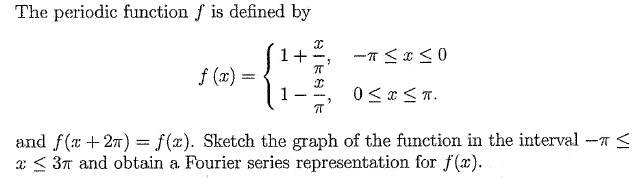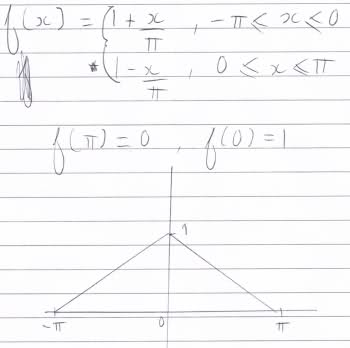# Short Fourier series question

## Homework Statement## Homework Equations

Usual equations for calculating fourier series coefficients

## The Attempt at a Solution

Well essentially I don't know what to let f(x) equal to for calculating the coefficients a0, an and bn. Should I use 1 + x/pi or 1 - x/pi? And what about the limits? I was thinking maybe between -pi and pi.

Anyway here's my progress thus far, I think the graph is ok anyways.Thanks dudes. I would use latex but I suck at it :yuck:

## Answers and Replies

I like Serena
Homework Helper
Looks fine.
Just start with the generic formula for a0.
The integral can be calculated by splitting it into the sum of 2 integrals.

Take for example:

$$a_1=\frac{1}{\pi}\int_{-\pi}^{\pi} f(x)\cos(x)dx$$

now, I want to integrate that function between -pi and pi but it's defined differently in two intervals. Why not just split up the intervals and write:

$$a_1=\frac{1}{\pi}\left(\int_{-\pi}^{0} (1+x\pi)\cos(x)dx+\int_{0}^{\pi} (1-x\pi)\cos(x)dx\right)$$

However it is an even function so there are short-cuts for computing them. But for now, you may want to just do it this way.

Take for example:

$$a_1=\frac{1}{\pi}\int_{-\pi}^{\pi} f(x)\cos(x)dx$$

now, I want to integrate that function between -pi and pi but it's defined differently in two intervals. Why not just split up the intervals and write:

$$a_1=\frac{1}{\pi}\left(\int_{-\pi}^{0} (1+x\pi)\cos(x)dx+\int_{0}^{\pi} (1-x\pi)\cos(x)dx\right)$$

However it is an even function so there are short-cuts for computing them. But for now, you may want to just do it this way.

Looks fine.
Just start with the generic formula for a0.
The integral can be calculated by splitting it into the sum of 2 integrals.

Great I understand completely now, thanks everyone.